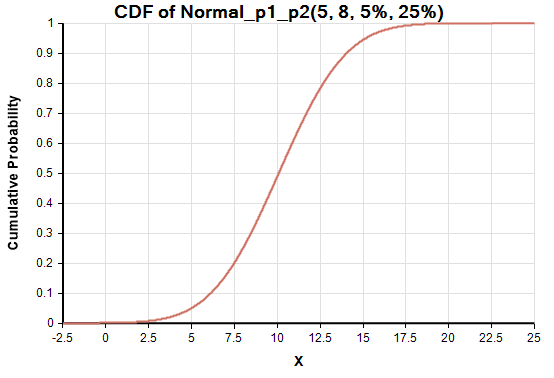# Normal p1 p2

New in Analytica 6.0

## Normal_p1_p2( q1, q2, p1, p2 )

This distribution function found in the Distribution Variations library defines a Normal distribution given any two percentile estimates. «q1» and «q2» are the percentile estimates, and «p1» and «p2» specify which percentiles these are. It must be that $\displaystyle{ q1\lt q2 }$ and $\displaystyle{ p1\lt p2 }$.

For example, given that the 5% percentile is 5 and the 25% percentile is 8, the distribution is

Normal_p1_p2( 5, 8, 5%, 25% ) →As with other distribution functions, you can also use this distribution in the function Random.

## Library

To use this function, you must first use Add Library to add the Distribution Variations Library to your model.